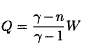Courses

# Thermodynamics - 2

## 20 Questions MCQ Test GATE Mechanical (ME) 2022 Mock Test Series | Thermodynamics - 2

Description
This mock test of Thermodynamics - 2 for GATE helps you for every GATE entrance exam. This contains 20 Multiple Choice Questions for GATE Thermodynamics - 2 (mcq) to study with solutions a complete question bank. The solved questions answers in this Thermodynamics - 2 quiz give you a good mix of easy questions and tough questions. GATE students definitely take this Thermodynamics - 2 exercise for a better result in the exam. You can find other Thermodynamics - 2 extra questions, long questions & short questions for GATE on EduRev as well by searching above.
QUESTION: 1

### A new temperature scale in degrees N is to be defined. The boiling and freezing points of water on this scale are 400° N and 100° N, respectively. What will be the reading on new scale corresponding to 60° C?

Solution: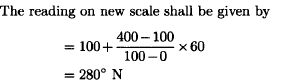QUESTION: 2

### Measurement of temperature is based on which law of thermodynamics?

Solution:

Zeroth law of thermodynamics states that if a body A is in thermal equilibrium with body B and body C, then the body B and body C are also in thermal equilibrium with each other. This law is specifically applied in temperature measurements.

QUESTION: 3

### A steam turbine receives steam steadily at 10 bar with an enthalpy of 3000 kj/kg and discharges at 1 bar with an enthalpy of 2700 kj/kg. The work output is 250 kJ/kg. The changes in kinetic and potential energies are negligible. The heat transfer from the turbine casing to the surroundings is equal to

Solution:

Given that
h1= 3000 kJ/kg

h2 = 2700 kJ/kg

W = 350 kJ/kg
Using steady state energy equation, h1 = h+ W + Q

3000 = 2700 + 250 + Q

Q = 50 kJ

QUESTION: 4

A system undergoes a change of state during which 80 kJ of heat is transferred to it and it does 60 kj of work. The system is brought back to its original state through a process during which 100 kj of heat is transferred to it. The work done by the system is

Solution:

For  the first process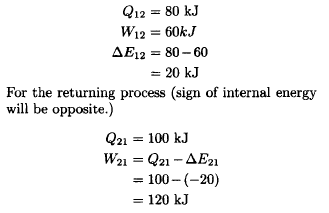QUESTION: 5

The internal energy of a certain system is a function of temperature alone and is given by the formula

E = 25 + 0.25t kJ

If this system executes a process for which the work done by it per degree temperature increase is 0.75 kNm, the heat interaction per degree temperature increase in kJ is

Solution:

Given that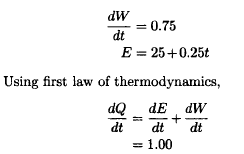QUESTION: 6

The air with enthalpy of 100 kJ/kg is compressed by an air compressor to a pressure and temperature at which its enthalpy becomes 200 kJ/kg. The loss of heat is 40 kJ/kg from the compressor as the air passes through it. Neglecting the change in kinetic and potential energies, the power required for an air mass flow of 0.5 kg/s is

Solution:

For given condition of air compressor steady flow of the energy equation is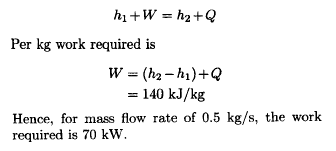QUESTION: 7

The cyclic integral of (SQ — 6W) for a process is

Solution:

According to the first law of thermodynamics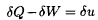where in intrenal energy u is the property of the system

QUESTION: 8

Suppose 0.70 kg/s of air enters the compressor with a specific enthalpy of 290 kJ/kg and leaves it with specific enthalpy 450 kJ/kg. Velocities at inlet and exit are 6 m/s and 2 m/s, respectively. Assuming adiabatic process, what is power input to the compressor?

Solution: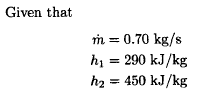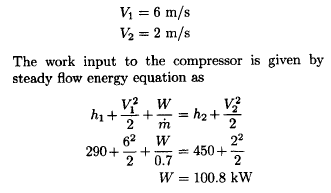QUESTION: 9

In a throttling process, which one of the following parameters remain constant?

Solution:

The enthalpy of the fluid before throttling is equal to the enthalpy of the fluid after throttling. The drop in pressure is compensated by corresponding change in density or internal energy of the flowing fluid.

QUESTION: 10

A refrigerating machine working on reversed Carnot cycle takes out 2 kW per minute of heat from the system while between temperature limits of 300 K and 200 K. COP and power consumption of the cycle will be respectively

Solution:

Given that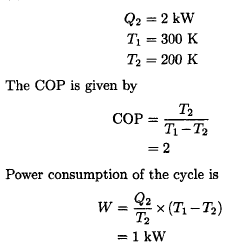QUESTION: 11

A reversible engine operates between temperatures T1 and T2. The energy rejected by this engine is received by a second reversible engine at temperature T2 and rejected to a reservoir at temperature T3. If the efficiencies of the engines are the same then the relationship between T1, T2 and T3 is given by

Solution: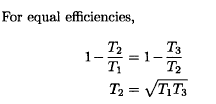QUESTION: 12

A Carnot engine operates between 327° C and 27° C. If the engine produces 300 kJ of work, what is the entiopy change during head addition?

Solution:

Given that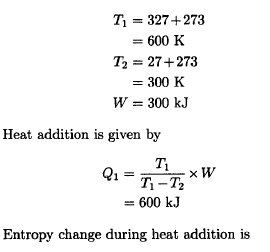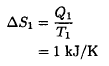QUESTION: 13

The relation

ds = dQ/T

where s represents entropy, Q represents heat and T represents temperature (absolute), holds good in which one of the following processes?

Solution:
QUESTION: 14

An inventor says that his new concept of an engine, while working between temperature limits of 27° C and 327° C rejects 45% of heat absorbed from the source. His engine is then equivalent to which one of the following engines?

Solution:

The efficiency of any reversible engine cannot be more than that of Carnot cycle. For the given case, the Carnot cycle efficiency is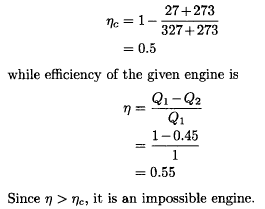QUESTION: 15

Three engines A, B and C operating on Carnot cycle use working substances as argon, oxygen and air, respectively. Which will have higher efficiency?

Solution:

The efficiency of reversible engine is independent of the working fluid

QUESTION: 16

A series of combination of two Carnot engines operate between the temperatures of 180° C and 20°C. If the engine produces equal amount of work, then what is the intermediate temperature?

Solution:

For equal amount of work, the intermediate temperature will be given as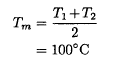QUESTION: 17

An engine working on Carnot cycle rejects 40% of absorbed heat from the source, while the sink temperature is maintained at 27°C, then what is the source temperature?

Solution: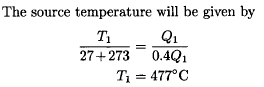QUESTION: 18

A reversible heat engine rejects 50% of the heat supplied during a cycle of operation. If this engine is reversed and operates as a heat pump, then what is its coefficient of performance?

Solution:

The efficiency of the given reversible heat engine is η = 0.5. The COP of the heat pump from this reversible cycle will be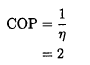QUESTION: 19

When the lower temperature is fixed, COP of a refrigerating machine can be improved by

Solution:

The performance parameter for a refrigerator cycle, called the coefficient of performance (COP), is defined as the ratio of desired thermal effect and work input to the system. It is expressed in terms of lower and higher temperatures as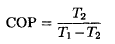Hence, COP can be increased by reducing higher temparature

QUESTION: 20

The heat absorbed or rejected during a polytropic process is equal to

Solution:

Heat transfer Q in a polytropic process can be represented in terms of work transfer W as